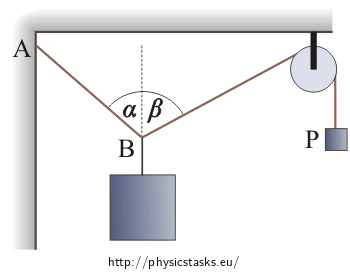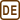## Weight on rope

Weight B with mass of 10 kg is fastened to a rope, as shown in the Figure. The distance AB is constant. How heavy weight P is it necessary to choose for α = 45°, β = 60°? How big will the stress be in rope AB in this case?• #### Notation

 mb = 10 kg mass of weight B α = 45° desired angle between rope AB and the vertical line β = 60° desired angle between the rope from B to the pulley and the vertical line mp =? (kg) mass of weight P Fa =? (N) stress of rope AB
• #### Hint 1

Draw all the forces acting on the weights B and P in the figure. What must be valid for their resultant?
• #### Hint 2

Choose an appropriate coordinate system and rewrite the equations (1) and (2) as a scalar. Express the searched parameters from the obtained equations.

• #### Solution

We draw all the forces acting on the weights B and P to the figure and choose the coordinate system.Weight P:

• $$\vec{F}_{Gp}$$ .......weight force (FGp = mpg)

• $$\vec{F}_{p}$$.......force, by which the rope is pulled

Weight B:

• $$\vec{F}_{Gb}$$ .......weight force (FGb = mbg)

• $$\vec{F^,}_{p}$$ ....... force, which the rope with the weight P is pulled by

(The pulley is at standstill so that it is valid for the values of forces $$\vec{F}_{p}$$ and $$\vec{F^,}_{p}$$ that Fp = F´p, The weight P pulls the rope with the weight B and vice versa)

• $$\vec{F}_{a}$$ ....... force, by which the rope mounted to the wall is pulled

Both weights are at standstill so the resultant of acting forces must be equal to the zero vector.

Weight P:

$\vec{F}_{Gp}+\vec{F}_{p}=\vec{0}\,.\tag{1}$

Weight B:

$\vec{F}_{Gb}+\vec{F'}_{p}+\vec{F}_{a}=\vec{0}\,.\tag{2}$

We rewrite the equations (1) and (2) as a scalar:

Weight P:

${F}_{p}=F_{Gp}=m_pg\,.$

Weight B:

$x:\hspace{60px}F_p\sin\beta-F_a\sin\alpha=0\tag{3}$

$y:\hspace{10px}F_a\cos\alpha+F_p\cos\beta-F_{Gb}=0\tag{4}$

From equation (3):

$F_a = F_p \frac{\sin \beta}{\sin \alpha}\,.\tag{5}$

We substitute into the equation (4):

$F_p \frac{\sin \beta}{\sin \alpha} \cos \alpha + F_p \cos \beta = F_{Gb}\,.$

And modify:

$F_p\frac{\sin\beta\cos\alpha+\sin\alpha\cos \beta}{\sin \alpha} = F_{Gb}\,.$

Using the addition formula for sine:

$F_p\sin(\alpha+\beta)=F_{Gb}\sin\alpha\,,$ $F_p=F_{Gb}\frac{\sin\alpha}{\sin(\alpha+\beta)}\,.$

So:

$gm_p=gm_b\frac{\sin\alpha}{\sin(\alpha+\beta)}\,,$ $m_p=m_b\frac{\sin\alpha}{\sin(\alpha+\beta)}\,.$

We substitute gained Fp to the equation (5):

$F_a = F_{Gb} \frac{\sin \alpha}{\sin (\alpha + \beta)} \frac{\sin \beta}{\sin \alpha}\,,$ $F_a = F_{Gb} \frac{\sin \beta}{\sin (\alpha + \beta)}\,,$ $F_a = gm_{b} \frac{\sin \beta}{\sin (\alpha + \beta)}\,.$

We substitute given values:

α, β, mb:

$m_p=10\frac {\sin45^\circ}{\sin(45^\circ+60^\circ)}\,\mathrm{kg}\dot{=}7{,}3 \,\mathrm{kg}\,,$ $F_a=10{\cdot}10\frac {\sin60^\circ}{\sin(45^\circ+60^\circ)}\,\mathrm{N} \dot{=} 90 \,\mathrm{N}\,.$

Demanded mass of weight P is equal to:

$m_p=m_b\frac{\sin\alpha}{\sin(\alpha+\beta)}\,.$

The stress in rope AB is equal to:

$F_a = gm_{b} \frac{\sin \beta}{\sin (\alpha + \beta)} \,.$

Numerically:

$m_p \dot{=}7.3 \,\mathrm{kg}\,,$ $F_a \dot{=} 90 \,\mathrm{N}\,.$